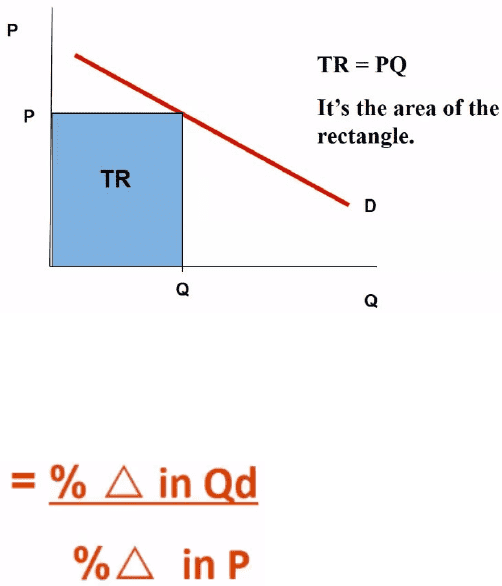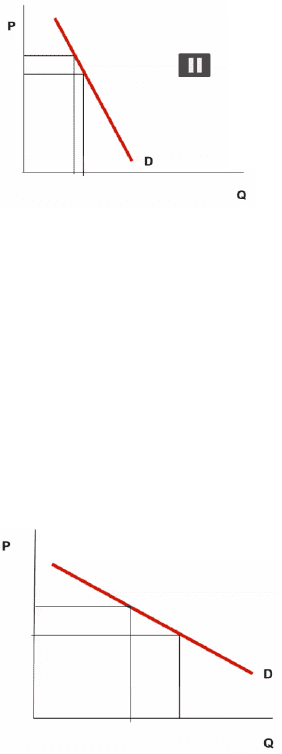Textbook Notes (280,000)
CA (160,000)
McMaster (10,000)
ECON (800)
ECON 1B03 (300)
Chapter 4

# ECON 1B03 Chapter Notes - Chapter 4: Demand Curve, Ice Cream Cone, HyperbolaPremium

Department
Economics
Course Code
ECON 1B03
Professor
Hannah Holmes
Chapter
4

This preview shows pages 1-2. to view the full 6 pages of the document.Price Elasticity of Demand
Elasticity
Measures the responsiveness of quantity demanded or quantity supplied is to changes
in price or other determinants
If price changes, will quantity demanded change by a little, or a lot
Important for firms to know how to maximize sales
Total revenue
Total Revenue = Price x Quantity Sold
Graph
Price elasticity of demand
How much the quantity demanded of a good responds to a change in the price of that
good
Equation
Ep = % change in quantity demanded / % change in Price
The result is
The coefficient of elasticity
How responsive demand is to a change in price
The larger the coefficient, the more responsive demand is
Types of price elasticity of demand
Inelastic demand
A change in price leads to a proportionately smaller change in quantity
demanded
Demand is not very responsive
Percentage change in price is greater than the percentage change in
quantity demanded
Ep < 1
Demand curve will be fairly steep

Only pages 1-2 are available for preview. Some parts have been intentionally blurred.

Subscribers OnlyPerfectly inelastic demand
Demand is not responsive at all to a price change
% change in quantity demanded will be 0
Ep = 0
Demand curve will be perfectly vertical
Elastic demand
Change in price leads to proportionately larger change in quantity
demanded
Demand is very responsive to a price change
Ep > 1
Demand curve will be fairly flat
Perfectly elastic demand
Change in price leads to and infinitely great change in quantity demanded
Demand is extremely responsive to a price change
Percentage change in quantity demanded approaches infinity
Ep = infinity
Demand curve will be horizontal
There are no real world examples
Unit elastic
Same change in price and quantity demanded
Ep = 1
Demand curve will be non linear
Total revenue must always be the same therefore is will be a
rectangular hyperbola